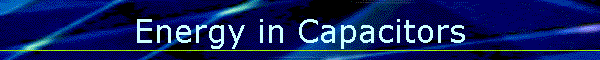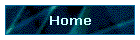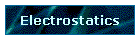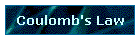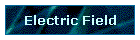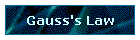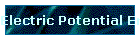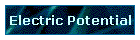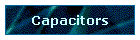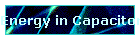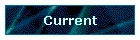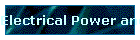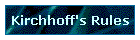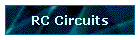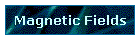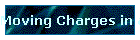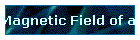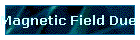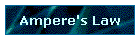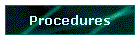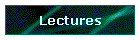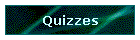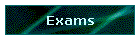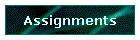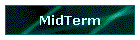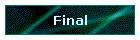Phys 4, Section 2                                                                    Feb 1, 2002  Energy Storage in Capacitors V = Q/C and dW = v dq = q dq/C. So the work W to charge a capacitor C to Q is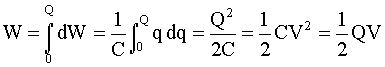Electric Field Energy If the work done in charging the capacitor is viewed as producing energy, this energy can be seen as stored in the capacitor (U = CV2/2) or in the electric field. If the energy U is divided by the volume Ad of space between the capacitor plates, then the space can be considered to have an energy density u given by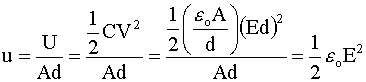Last modified on February 05, 2002